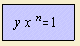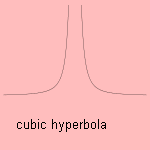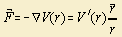# polytrope

## higherA polytrope 1) has two asymptotes, and is either symmetric round the y-axis (even n), either symmetric round zero (odd n).
Some special cases:Polytropic equations are used by nature for a variety of forces: the force is inversely proportional to an integer power of the distance from the source. Each force is related to a so-called potential, described by a polytrope with a degree of one more 2).

Best known cases are gravitation and the electrostatic force. The force is inversely proportional to the square distance. This relation reminds us of phenomena as the decreasing of light and sound with distance from the source. The propagating oscillation fills a whole area's sphere, and that area is proportional to the square of the radius.

There are other also polytropic forces that fall down faster with the distance. Those are forces that work on an atomic scale.

When we consider an ideal (inert) gas, we see an attraction between the atoms, as result of the dipole nature of the atoms. It can be derived that this so called van der Waals interaction 3) has a potential that is inversely proportional to the sixth power of the distance.

Equilibrium in this situation is settled by another, yet a repulsive power. This power works as result of the overlap of electron tracks. Experimental has been settled that this potential is inversely proportional to the twelfth power of the distance.

In physiology the Gorlin formula gives the relation between the blood flow F and the pressure gradient p as p√F = C, thus giving a cubic hyperbolic relation.

The softer sciences encounter polytropes too. There has been an investigation how the year's numbers in a newspaper are distributed 4). It occurred that there was a difference between events before and after 1910. Before this year (about the length of a man's life ago) the events extinguish inversely to the square of the passed time, after that year inversely to the passed time. What do you think, is this science, or is this an invention of a relation that is not there?

notes

1) Poly = strong, tropč = turn.

2) As we know for the relation between potential and force:3) Consider the atoms as harmonic vibrating dipoles, Kittel 1976 p.78

4) Pollmann 1996